RS Aggarwal Class 6 Solutions Chapter 4 - Integers

RS Aggarwal Class 6 Chapter 4 - Integers Solutions Free PDF

The Chapter 4 – Integers for Class 6 explains the definition of the concept integers, types of integers, multiplication, subtraction, multiplication and division of integers. An integer is a whole number that can be positive, negative or zero. To master the concepts of integers students are advised to solve the questions from RS Aggarwal for Class 6 and for the solution to those questions they can to refer RS Aggarwal Class 6 solutions Chapter 4 – Integers.

At BYJU’S we have provided all the solutions of RS Aggarwal Maths for Class 6 to help you revise the complete syllabus and score good marks. Making the study materials readily available will make the preparation for exams mare easier for students. Practicing these solutions helps them in comprehensively covering all topics and also find out topics they are weak in and needs extra attention.

Question 1:

Write the opposite of each of the following:

(i) An increase of 8

(ii) A loss of Rs. 7

(iii) Gaining a weight of 5 kg

(iv) 10 km above sea level

(v) 5oC below the freezing point

(vi) A deposit of Rs. 100

(vii) Earning Rs. 500

(viii) Going 6 m to the east

(ix) 24

(x) -34

Solution:

(i) A decrease of 8.

(ii) A gain of Rs. 7.

(iii) Losing a weight of 5 kg.

(iv) 10 km below the sea level.

(v) 5oC above the freezing point..

(vi) A withdrawal of Rs. 100.

(vii) Spending Rs. 500.

(viii) Going 6 m to the west.

(ix) -24.

(x) 34.

Question 2:

Indicate the following using ‘ + ’ or ‘ – ’ sign:

(i) A gain of Rs. 600

(ii) A loss of Rs. 800

(iii) 7oC below the freezing point

(iv) Decrease of 9

(v) 2 km above sea level

(vi) 3 km below sea level

(vii) A deposit of Rs. 200

(viii) A withdrawal of Rs. 300

Solution:

(i) + Rs. 600

(ii) – Rs. 800

(iii) -7oC

(iv) -9

(v) +2 km

(vi) -3 km

(vii) + Rs. 200

(viii) – Rs. 300

Question 3:

Mark the following integers on a number line:

(i) -5

(ii) -2

(iii) 0

(iv) 7

(v) -13

Solution:

(i) -5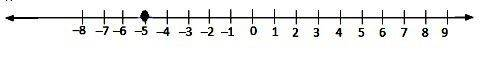(ii) -2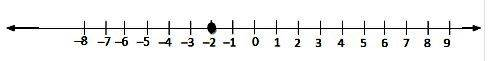(iii) 0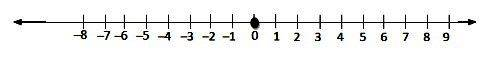(iv) 7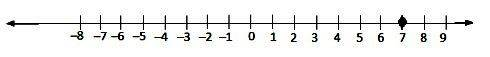(v) -13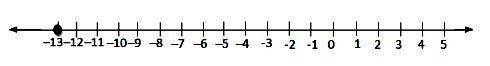Question 4:

Which number is larger in each of the following pairs?

(i) 0, -2

(ii) -3, -5

(iii) -5, 2

(iv) -16, 8

(v) -365, -913

(vi) -888, 8

Solution:

(i) 0, -2

0 > -2

This is because zero is greater than every negative integer.

(ii) -3, -5

-3 > -5

Since 3 is smaller than 5, -3 is greater than -5

(iii) -5, 2

2 > -5

This is because every positive integer is greater than every negative integer.

(iv) -16, 8

8 > -16

This is because every positive integer is greater than every negative integer.

(v) -365, -913

-365 > -913

Since 365 is smaller tha 913, -365 is greater than -913.

(vi) -888, 8

8 > -888

This is because every positive integer is greater than every negative integer.

Question 5:

Which number is smallerin each of the following pairs?

(i) 6, -7

(ii) 0, -1

(iii) -13, -27

(iv) -26, 17

(v) -317, -603

(vi) -777, 7

Solution:

(i) -7 < 6

This is because every positive integer is greater than every negative integer.

(ii) -1 < 0

This is because zero is greater than every negative integer.

(iii) -27 < -13

Since 27 is greater than 13, -27 is smaller than -13.

(iv) -26 < 17

This is because every positive integer is greater than every negative integer.

(v) -603 < -317

Since 603 is greater than 317, -603 is smaller than -317.

(vi) -777 < 7

This is because every positive integer is greater than every negative integer.

Question 6:

Write all integers between

(i) 0 and 6

(ii) -5 and 0

(iii) -3 and 3

(iv) -7 and -5

Solution:

(i) 1, 2, 3, 4, 5.

(ii) -4, -3, -2, -1

(iii) -2, -1, 0, 1, 2

(iv) -6

Question 7:

Fill in the blanks by appropriate symbol > or <:

(i) 0 ………. 7

(ii) 0 ………. -3

(iii) -5 ………. -2

(iv) -15 ………. 13

(v) -231 ………. -132

(vi) -6 ………. 6

Solution:

(i) 0 < 7

This is because 0 is less than any positive integer.

(ii) 0 > -3

This is because 0 is greater than any negative integer.

(iii) -5 < -2

Since 5 is greater than 2, -5 is smaller than -2.

(iv) -15 < 13

This is because every positive integer is greater than every negative integer.

(v) -231 < -132

Since 231 is greater than 132, -231 is smaller than -132.

(vi) -6 < -6

This is because every positive integer is greater than every negative integer.

Question 8:

Write the following integers in the increasing order:

(i) 5, -7, -2, 0, 8

(ii) -23, 12, 0, -6, -100, -1

(iii) -17, 15, -363, -501, 165

(iv) 21, -106, -16, 16, 0, -2, -81

Solution:

(i) -7 < -2 < 0 < 5 < 8

(ii) -100 < -23 < -6 < -1 < 0 < 12

(iii) -501 < – 363 < -17 < 15 < 165

(iv) -106 < -81 < -16 < -2 < 0 < 16 < 21

Question 9:

Write the following integers in the decreasing order:

(i) 0, 7, -3, -9, -132, 36

(ii) 51, -53, -8, 0, -2

(iii) -71, -81, 36, 0, -5

(iv) -365, -515, 102, 413, -7

Solution:

(i) 36 > 7 > 0 > -3 > -9 > – 132

(ii) 51 > 0 > -2 > -8 > -53

(iii) 36 > 0 > -5 > -71 > -81

(iv) 413 > 102 > -7 > -365 > 515

Question 10:

Using the number line, write the integer which is

(i) 4 more than 6

(ii) 5 more than -6

(iii) 6 less than 2

(iv) 2 less than -3

Solution:

(i) 4 more than 6

We want an integer that is 4 more than 6. So, we will start from 6 and proceed 4 steps to the right to obtain 10.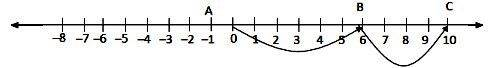(ii) 5 more than -6

We want an integer that is 5 more than -6. So, we will start from -6 and proceed 5 steps to the right to obtain -1.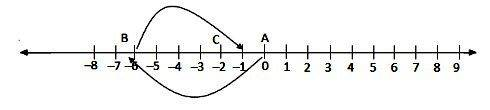(iii) 6 less than 2

We want an integer that is 6 less than 2. So, we will start from 2 and proceed 6 steps to the left to obtain -4.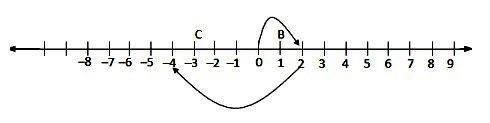(iv) 2 less than -3

We want an integer that is 2 less than -3. So, we will start from -3 and proceed 2 steps to the left to obtain -5.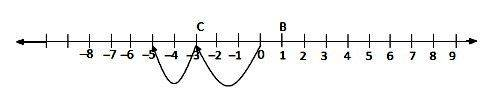Question 11:

For each of the following statements, write (T) for true and (F) for false:

(i) The smallest integer is zero.

(ii) Zero is not an integer.

(iii) The opposite of zero is zero.

(iv) -10 is greater than -6

(v) The absolute value of an integer is always greater than the integer.

(vi) 0 is larger than every negative integer.

(vii) Every negative integer is less than every natural number.

(viii) The successor of -187 is -188.

(ix) The predecessor of -215 is -214.

Solution:

(i) False

This is because 0 is greater than every negative integer.

(ii) False

0 is an integer as we know that every whole number is an integer and 0 is a whole number.

(iii) True

0 is an integer that is neither positive nor negative. So, the opposite of zero is zero.

(iv) False

Since 10 is greater than 6, -10 is smaller than -6.

(v) True

This is because an absolute value is a positive number. For example, -2 is an integer, but its absolute value is 2 and it is greater than -2.

(vi) True

This is because all negative integers are to the left of 0.

(vii) True

This is because natural numbers are positive and every positive integer is greater than every negative integer.

(viii) False

This is because the successor of -187 is equal to -186 (-187 + 1). In succession, we move from the left to the right along a number line.

(ix) False

This is because the predecessor of -215 is -216 (-215 – 1). To find the predecessor, we move from the right to the left along a number line.

Question 12:

Find the value of

(i) |-9|

(ii) |-36|

(iii) |0|

(iv) |15|

(v) -|-3|

(vi) 7 + |-3|

(vii) |7 – 4|

(viii) 8 – |-7|

Solution:

(i) The value of |-9| is 9.

(ii) The value of |-36| is 36.

(iii) The value of |0| is 0.

(iv) The value of |15| is 15.

(v) The value of |-3| is -3.

(vi) 7 + |-3|

= 7 + 3 = 10

(vii) |7 – 4|

= |3| = 3

(viii) 8 – |-7|

= 8 – 7 = 1

Question 13:

(i) Write five negative integers greater than -7.

(ii) Write five negative integers less than -20.

Solution:

(i) Every negative integer that is to the right of -7 is greater than -7.

So, five negative integers that are greater than -7 are -6, -5, -4, -3, -2 and -1.

(ii) Every negative integer that is to the left of -20 is less than -20.

So, five negative integers that are less than -20 are -21, -22, -23, -24 and -25.

RS Aggarwal Class 6 Solutions Chapter 4 – Integers

The RS Aggarwal Maths Solutions are considered an extremely helpful resource for exam preparation. The questions provided in the RS Aggarwal reference textbook are prepared according to CBSE, thus holding higher chances of appearing on CBSE question papers. It helps students to tackle difficult types of question easily. The solutions included are easy to understand, and each step in the solution is described to match the student’s understanding. The solutions for difficult questions are available in an easy form, you can practice the great number of questions before the exam to get enhance your question-solving skills.

Practise This Question

What type of motion is represented by the tip of second hand of a clock?